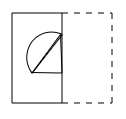top of page
Search
• APSEd

# Spatial Aptitude | Mirror Image and Paper folding

In spatial aptitude, many topics like mirror images, paper folding and paper cutting are there. Here, we will see in detail about mirror images and paper folding. According to the updated syllabus of GATE 2021, one of the topics is spatial aptitude.The mirror image of an object about a line which is called a mirror line. It can be easily obtained by just inverting the object about the mirror line without disturbing the relative positions of the control points of the object. The control points of an object reference to its corners, midpoints, etc., which would make the inversion of the object easier.

The concept of mirror image can be understood easily if you imagine as if a mirror is placed on the mirror line. The following 2 points should always be satisfied while making mirror images. They are:

• Every point of the object and its corresponding point in the mirror image should at the same distance from the mirror line.

• The mirror image should be of the same size as the original object.

Now, let's see an example to understand the concept of mirror image more clearly.For this figure given, if we place a mirror below this figure, the mirror image obtained about the mirror image is shown below.The important points to be noted is that all points of the original figure and the mirror image are equidistant from the mirror line. Also, if you try to superimpose the mirror image after inversion about the mirror line, both the original and mirror images would be overlapping and you would obtain a single image.

If you practice some questions from previous year papers of SSC, SBI PO and banking exams, you would get a better understanding of this topic.

Now, let's see about paper folding.

## Paper folding

In questions related to paper folding, a transparent sheet of paper with designs on it should be imagined and will be folded in 2-3 steps according to the folding steps given in the question. The options of the question will be the figure which will be obtained after we will fold the sheet completely and how the designs would be observed.

Let's see an example to understand this concept clearly. The question will be to find out as to how the pattern would appear when the transparent sheet is folded at the dotted line.In the above figure, a design with a triangle and a semicircle is given. The dotted line represents the line about which the paper would be folded so that and after folding how the design appears is the answer to the question. The folded paper design to the figure is given below.The dotted line is occurring on the right side of the sheet which means that the sheet has been folded from the right to the left. After folding, The triangle on the right side of the dotted middle line will get overlapped on the left triangle and the semicircle remains as such on the left side of the paper.

Similar to mirror images, by practising some questions, these topics will help you to improve your score in exams. These type of questions are regularly asked in PSU exams so learning these topics thoroughly can help you to score more marks in technical exams other than GATE also.

Try this question out!

Subscribe to APSEd Blog and get to know the latest.